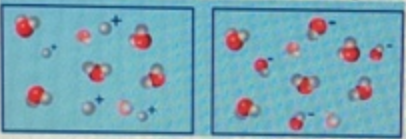# Problem: How does the pH of the solution on the right compare with that of the solution on the left? a) The solution on the right is acidic relative to the solution on the left.b) The solution on the right is basic relative to the solution on the left. c) Both of these solutions are equally basic.d) The solution on the right is neutral relative to the solution on the left.e) Both of these solutions are equally acidic.

###### FREE Expert Solution

Recall: pH = –log [H+] or [H3O+]

Also recall, ↑ H+ = ↓ pH (acidic) and ↑ OH = ↑ pH (basic)###### Problem Details

How does the pH of the solution on the right compare with that of the solution on the left?a) The solution on the right is acidic relative to the solution on the left.

b) The solution on the right is basic relative to the solution on the left.

c) Both of these solutions are equally basic.

d) The solution on the right is neutral relative to the solution on the left.

e) Both of these solutions are equally acidic.